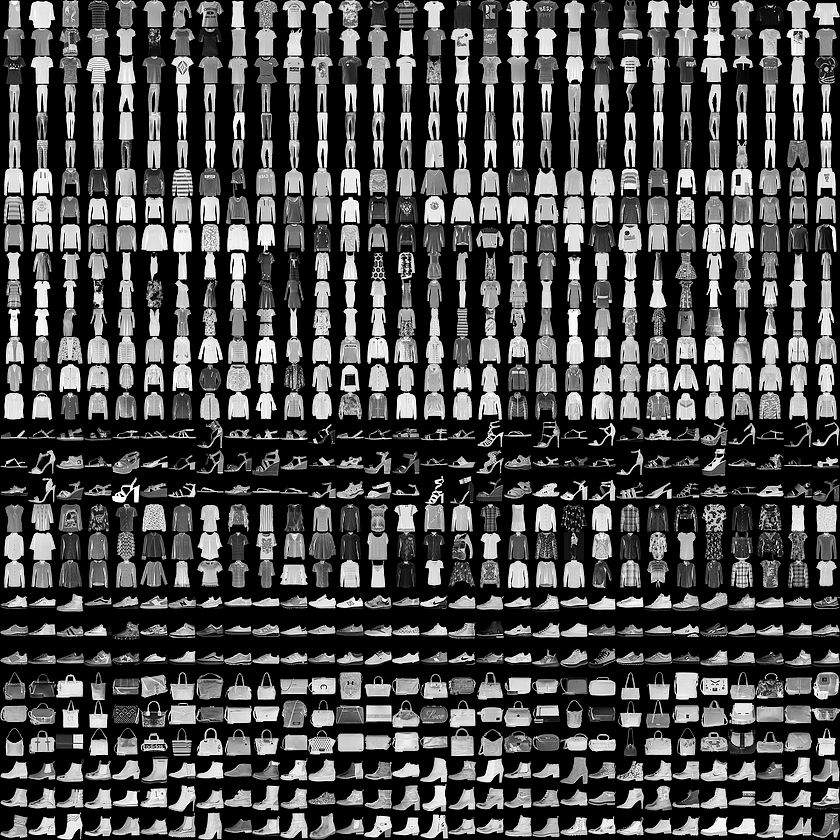1. 训练您的第一个神经网络：基本分类Fashion MNIST(tensorflow2.0官方教程翻译)

# 训练您的第一个神经网络：基本分类Fashion MNIST(tensorflow2.0官方教程翻译)

pip install tensorflow==2.0.0-alpha0


from __future__ import absolute_import, division, print_function, unicode_literals

# TensorFlow and tf.keras
import tensorflow as tf
from tensorflow import keras

# Helper libraries
import numpy as np
import matplotlib.pyplot as plt

print(tf.__version__)


## 1. 导入MNIST数据集Figure 1. Fashion-MNIST 样本

Fashion MNIST 的作用是成为经典 MNIST 数据集的简易替换，后者通常用作计算机视觉机器学习程序的“Hello, World”入门数据集。MNIST数据集包含手写数字（0、1、2 等）的图像，这些图像的格式与我们在本教程中使用的服饰图像的格式相同。

fashion_mnist = keras.datasets.fashion_mnist

(train_images, train_labels), (test_images, test_labels) = fashion_mnist.load_data()


• train_imagestrain_labels数组是训练集，即模型用于学习的数据。
• 测试集 test_imagestest_labels 数组用于测试模型。

Label Class
0 T-shirt/top(T 恤衫/上衣)
1 Trouser(裤子)
2 Pullover (套衫)
3 Dress(裙子)
4 Coat(外套)
5 Sandal(凉鞋)
6 Shirt(衬衫)
7 Sneaker(运动鞋)
8 Bag(包包)
9 Ankle boot(踝靴)

class_names = ['T-shirt/top', 'Trouser', 'Pullover', 'Dress', 'Coat',
'Sandal', 'Shirt', 'Sneaker', 'Bag', 'Ankle boot']


## 2. 探索数据

train_images.shape


(60000, 28, 28)

len(train_labels)


60000

train_labels


array([9, 0, 0, ..., 3, 0, 5], dtype=uint8)

test_images.shape


(10000, 28, 28)

len(test_labels)


10000

## 3. 预处理数据

plt.figure()
plt.imshow(train_images)
plt.colorbar()
plt.grid(False)
plt.show()train_images = train_images / 255.0

test_images = test_images / 255.0


plt.figure(figsize=(10,10))
for i in range(25):
plt.subplot(5,5,i+1)
plt.xticks([])
plt.yticks([])
plt.grid(False)
plt.imshow(train_images[i], cmap=plt.cm.binary)
plt.xlabel(class_names[train_labels[i]])
plt.show()## 4. 构建模型

### 4.1. 设置图层

model = keras.Sequential([
keras.layers.Flatten(input_shape=(28, 28)),
keras.layers.Dense(128, activation='relu'),
keras.layers.Dense(10, activation='softmax')
])


### 4.2. 编译模型

• 损失函数：衡量模型在训练期间的准确率。我们希望尽可能缩小该函数，以“引导”模型朝着正确的方向优化。
• 优化器：根据模型看到的数据及其损失函数更新模型的方式。
• 度量标准：用于监控训练和测试步骤。以下示例使用准确率，即图像被正确分类的比例。
model.compile(optimizer='adam',
loss='sparse_categorical_crossentropy',
metrics=['accuracy'])


## 5. 训练模型

1. 将训练数据馈送到模型中，在本示例中为 train_imagestrain_labels 数组。
2. 模型学习将图像与标签相关联。
3. 我们要求模型对测试集进行预测，在本示例中为 test_images 数组。我们会验证预测结果是否与 test_labels 数组中的标签一致。

model.fit(train_images, train_labels, epochs=5)

Epoch 1/5
60000/60000 [==============================] - 5s 87us/step - loss: 0.5033 - acc: 0.8242
......
Epoch 5/5
60000/60000 [==============================] - 5s 88us/step - loss: 0.2941 - acc: 0.8917


## 6. 评估精度

test_loss, test_acc = model.evaluate(test_images, test_labels)

print('\nTest accuracy:', test_acc)


10000/10000 [==============================] - 1s 50us/step
Test accuracy: 0.8734


## 7. 预测

predictions = model.predict(test_images)


predictions


array([6.2482708e-05, 2.4860196e-08, 9.7165821e-07, 4.7436039e-08,
2.0804382e-06, 1.3316551e-02, 9.8731316e-06, 3.4591161e-02,
1.2390658e-04, 9.5189297e-01], dtype=float32)


np.argmax(predictions)


9

test_labels


9

def plot_image(i, predictions_array, true_label, img):
predictions_array, true_label, img = predictions_array[i], true_label[i], img[i]
plt.grid(False)
plt.xticks([])
plt.yticks([])

plt.imshow(img, cmap=plt.cm.binary)

predicted_label = np.argmax(predictions_array)
if predicted_label == true_label:
color = 'blue'
else:
color = 'red'

plt.xlabel("{} {:2.0f}% ({})".format(class_names[predicted_label],
100*np.max(predictions_array),
class_names[true_label]),
color=color)

def plot_value_array(i, predictions_array, true_label):
predictions_array, true_label = predictions_array[i], true_label[i]
plt.grid(False)
plt.xticks([])
plt.yticks([])
thisplot = plt.bar(range(10), predictions_array, color="#777777")
plt.ylim([0, 1])
predicted_label = np.argmax(predictions_array)

thisplot[predicted_label].set_color('red')
thisplot[true_label].set_color('blue')


i = 0
plt.figure(figsize=(6,3))
plt.subplot(1,2,1)
plot_image(i, predictions, test_labels, test_images)
plt.subplot(1,2,2)
plot_value_array(i, predictions,  test_labels)
plt.show()i = 12
plt.figure(figsize=(6,3))
plt.subplot(1,2,1)
plot_image(i, predictions, test_labels, test_images)
plt.subplot(1,2,2)
plot_value_array(i, predictions,  test_labels)
plt.show()# 绘制前X个测试图像，预测标签和真实标签。
# 用蓝色标记正确的预测，用红色标记错误的预测。
num_rows = 5
num_cols = 3
num_images = num_rows*num_cols
plt.figure(figsize=(2*2*num_cols, 2*num_rows))
for i in range(num_images):
plt.subplot(num_rows, 2*num_cols, 2*i+1)
plot_image(i, predictions, test_labels, test_images)
plt.subplot(num_rows, 2*num_cols, 2*i+2)
plot_value_array(i, predictions, test_labels)
plt.show()# 从测试数据集中获取图像
img = test_images

print(img.shape)


tf.keras模型已经过优化，可以一次性对样本批次或样本集进行预测。因此，即使我们使用单个图像，仍需要将其添加到列表中：

# 将图像添加到批次中，它是唯一的成员。
img = (np.expand_dims(img,0))

print(img.shape)


(1, 28, 28)

predictions_single = model.predict(img)

print(predictions_single)

plot_value_array(0, predictions_single, test_labels)
_ = plt.xticks(range(10), class_names, rotation=45)model.predict返回一组列表，每个列表对应批次数据中的每张图像。（仅）获取批次数据中相应图像的预测结果：

np.argmax(predictions_single)


9